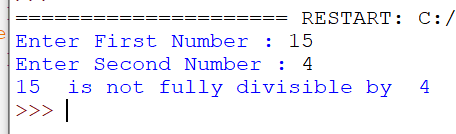# O Level Exam

#### Programming Example

WAP to take two numbers and check that the first number is fully divisible by second number or not.

`Solution`

```a=int(input("Enter First Number : "))
b=int(input("Enter Second Number : "))
if a%b==0:
print(a," is fully divisible by ",b)
else:
print(a," is not fully divisible by ",b)```
`Output`